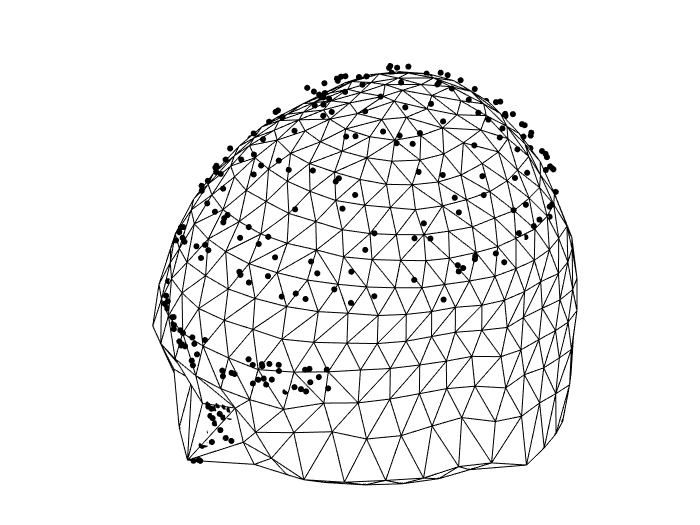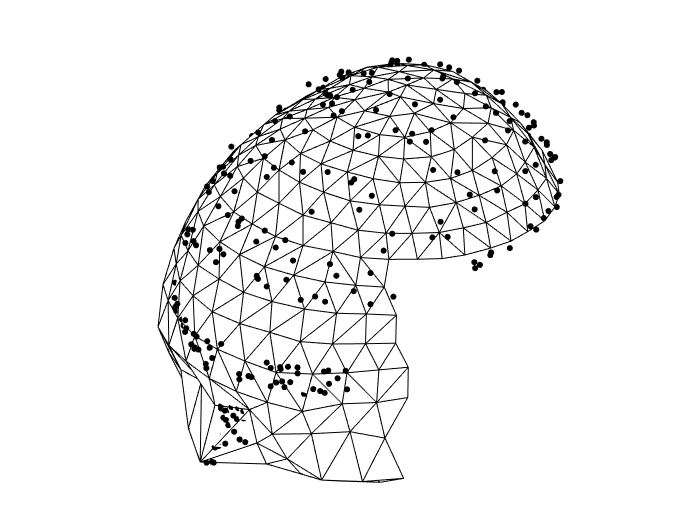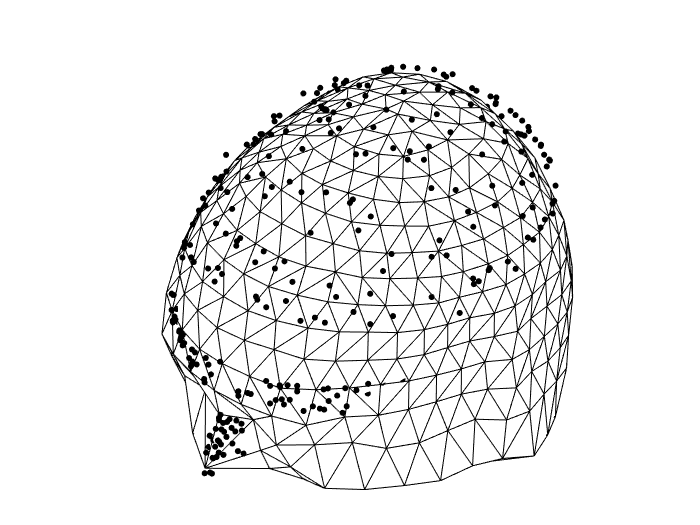Tags: example source

# How to create a head model if you do not have an individual MRI

## Introduction

A volume conduction model of the head is required for source reconstruction. Ideally you base the head model on the individual’s anatomical MRI, but that is not always available. In the case of EEG you can use a template head model and fit your measured electrodes (or template electrodes) on the scalp of the template model. For MEG you cannot simply use a template head model, since the distance between the head and the (fixed) MEG sensors depends on the head size.

In this example we will show a few ways on how to create an individual head model on the basis of surface data acquired with the Polhemus. We will determine the translation, rotation and scaling of the template head model to fit the Polhemus head shape.

These approaches will be demonstrated using the same dataset, which you can find on the download server. More information on this dataset can be found here.

You load the head shape measured during the MEG recording with the Polhemus and a template volume conduction model. We have to ensure that they have consistent units, hence we will convert the units into mm. Expressing the data in mm will give a better expression of the data (i.e. 90mm vs. 0.09m).

``````polhemus = ft_read_headshape('epilepsy/case1/ctf_data/case1.pos');
polhemus = ft_convert_units(polhemus, 'mm');

template = ft_convert_units(template, 'mm');
``````

Note that the template head model contains three surfaces describing the three compartments of scalp, skull and brain. Furthermore, it descibes the conductivities and the BEM system matrix, computed with dipoli. Here is how the complete structure looks like

``````template =
struct with fields:

bnd: [1x3 struct]
cond: [0.3300 0.0041 0.3300]
mat: [3000x3000 double]
type: 'dipoli'
unit: 'mm'

% note that the template's precomputed system matrix needs to be deleted
% because this gives problems with spatial transformations
template = rmfield(template, {'mat' 'type'});
``````

## Coregistration

In the next step we coregister the template head model with the Polhemus head shape to ensure that they are expressed consistently in the MEG coordinate system (i.e. relative to the same origin and with the axes pointing in the same direction). Normally the coregistration only consists of rotation and translation, but in this case you can also specify scaling.

``````cfg = [];
cfg.checksize               = inf;
cfg                         = ft_interactiverealign(cfg);
template                    = ft_transform_geometry(cfg.m, template);
``````

For the method that uses spheres to transform the template it is important to get the rotation correct, as spheres are not sensitive to rotations.

For the method that uses both surfaces to transform the template it is good enough to have an initial guess of the rotation.

In this step you can already accurately rotate, translate and scale the template head model to fit the Polhemus head surface and skip directly to compute the head model. However, this is time consuming and following methods should be adopted.

## Refining the transformation

### Method 1: On the basis of spheres fitted to the head shapes

We will fit a sphere to the scalp surface measured with the Polhemus and another sphere to the scalp surface of the template. On the basis of the two spheres we can derive a translation and global scaling. Important to note is that the template head model consists of three surfaces (template.bnd) describing the (1) scalp, (2) skull and (3) brain. Only the scalp surface will be used to determine the sphere.

Fit a sphere to the template scalp surface.

``````cfg             = [];
cfg.method      = 'singlesphere';
``````

Fit a sphere to the Polhemus scalp surface

``````cfg              = [];
cfg.method      = 'singlesphere';
``````

Determine the global scaling and translation

``````scale = sphere_polhemus.r/sphere_template.r;

T1 = [1 0 0 -sphere_template.o(1);
0 1 0 -sphere_template.o(2);
0 0 1 -sphere_template.o(3);
0 0 0 1                ];

S  = [scale 0 0 0;
0 scale 0 0;
0 0 scale 0;
0 0 0 1 ];

T2 = [1 0 0 sphere_polhemus.o(1);
0 1 0 sphere_polhemus.o(2);
0 0 1 sphere_polhemus.o(3);
0 0 0 1                 ];

transformation = T2*S*T1;
``````

Apply the transformation to the template head model

``````template_fit_sphere = ft_transform_geometry(transformation, template);
``````

### Method 2: On the basis of the full head surface

We determine an affine transformation that fits the template scalp surface to the Polhemus surface. This not only applies a translation and rotation, but also a scaling in the different directions and some skewing.

It is important that the template scalp surface only contains features that are also in the Polhemus surface, and vice versa. We can use ft_defacemesh to remove some features.

We visualize both meshes:

``````figure;
ft_plot_mesh(template.bnd(1));
ft_plot_mesh(polhemus);
``````The Polhemus has facial details which are not in the template scalp surface, and the Polhemus does not cover the lower back of the head. These details need to be removed.

``````defaced_template      = template.bnd(1);
defaced_template.unit = template.unit

cfg              = [];
cfg.translate    = [-40 0 -50];
cfg.scale        = [200 200 200];
cfg.rotate       = [0 0 0];
defaced_template =  ft_defacemesh(cfg, defaced_template);

cfg              = [];
cfg.translate    = [-40 0 -50];
cfg.scale        = [200 200 200];
cfg.rotate       = [0 0 0];
defaced_polhemus =  ft_defacemesh(cfg, polhemus);
``````

We have another look how well the surfaces match

``````figure;
ft_plot_mesh(defaced_template);
ft_plot_mesh(defaced_polhemus);
``````We determine the transformation and apply it to all 3 surfaces of the template head model.

``````cfg                  = [];
cfg.template         = defaced_template;
cfg.method           = 'fittemplate';
template_fit_surface = ft_prepare_mesh(cfg, template.bnd);
``````

We can compute the volume conduction model on the basis of the refined template head models. For this we need to specify conductivities for each compartment (scalp, skull and brain). We specify these conductivities in SI units. Therefore the refined models need to be expressed in SI units as well.

``````template_fit_sphere  = ft_convert_units(template_fit_sphere,'m');
template_fit_surface = ft_convert_units(template_fit_surface,'m');
``````

### Openmeeg

We create the volume conduction models using Openmeeg. This model can be later used for EEG or MEG volume conduction modeling.

``````cfg              = [];
cfg.conductivity = [0.33 0.0042 0.33];
cfg.method       = 'openmeeg';

figure;
ft_plot_mesh(polhemus)
````````````cfg               = [];
cfg.conductivity  = [0.33 0.0042 0.33];
cfg.method        = 'openmeeg';

figure;
ft_plot_mesh(polhemus)
``````### Taking the inner shell for a single-shell model

Another option for MEG is to create a single-shell model on the basis of the brain compartment.

``````cfg                          = [];
cfg.method                   = 'singlesphere';